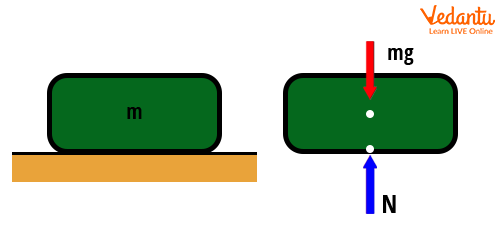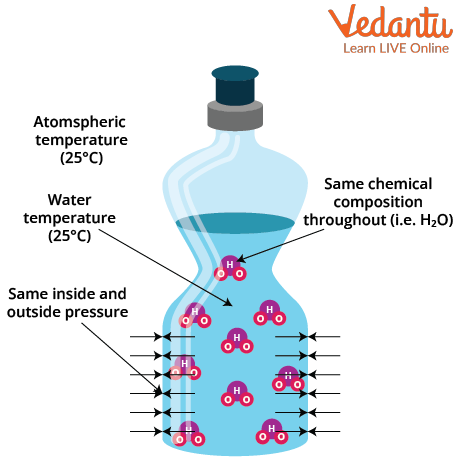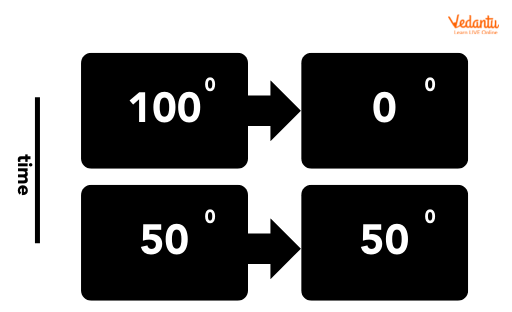Courses
Courses for Kids
Free study material
Free LIVE classes
More

# Thermal Equilibrium in Thermodynamics for JEELIVE
Join Vedantu’s FREE Mastercalss

## Thermal Equilibrium Definition

Thermodynamics is a macroscopic science, where we study the different forms of heat and the conversion of heat into other forms of energy in different ways. So, it deals with the bulk system and does not require the knowledge of molecular constitution of matter. In thermodynamics, the state of matter is specified by macroscopic variables such as temperature, pressure and volume.

In thermodynamic equilibrium, the state represents a system where the macroscopic variables that characterise the system do not vary with time. Consider a gas inside a closed and well-insulated container that has fixed values of pressure, volume, temperature, mass and composition, and these variables do not change with time. We can say that the gas inside the container is in the state of thermodynamic equilibrium. Now the unanswered question, what is thermal equilibrium? Thermal equilibrium is a particular type of equilibrium state in which no heat exchange occurs between various points in a system. Hence the temperature will be uniform everywhere. At thermal equilibrium, the heat and temperature are the main components studied.

## Types of Equilibrium in Thermodynamics

Equilibrium is a state in which the properties of the system are uniform everywhere and do not change with time.

1. Thermal Equilibrium: A system will be in thermal equilibrium when there is no heat transfer inside the system, and temperature is uniform throughout the system. For example: if we keep a glass of cool water at 10oC at room temperature say 25oC, after some time the water in the glass will attain room temperature 25oC. The heat transfer occurs between the system and the surroundings, till it reaches a thermal equilibrium.Example of Thermal Equilibrium

1. Mechanical Equilibrium: A system is said to be in mechanical equilibrium when the pressure is uniform everywhere in the system. For example, consider an object at rest. The normal force N is equal, opposite and collinear to the gravitational force mg, so the net force and moment is zero. Hence, the object is in a state of static mechanical equilibrium.An Object at Rest at Mechanical Equilibrium as Net Force Acting is Zero

1. Thermodynamic Equilibrium: A system where there is no heat or work, and no mass exchange occurs, is said to be in thermodynamic equilibrium. For example: A bottle of water kept at room temperature. The pressure inside which is exerted by water molecules is the same as outside pressure. Hence, the water in the bottle is at mechanical  equilibrium.

The composition of water inside the bottle is the same everywhere. Thus, it is at chemical equilibrium. Also, water temperature and surrounding temperature is the same as atmospheric temperature. So,  the water in the bottle is at thermal equilibrium. The system here obeys mechanical, thermal and chemical equilibrium. Hence it is in thermodynamic equilibrium.A Bottle of Water in Thermodynamic Equilibrium

## Mechanism of Thermal Equilibrium

Temperature represents the energy of molecules in a substance. It is the measure of average kinetic energy of atoms or molecules in a substance. Two objects at different temperatures will have molecules with different kinetic energies.  When these objects come into contact with one another, the molecules with more kinetic energy in one material will collide with the molecules with a lesser kinetic energy in the other.

This may lead the system to a state where both objects will have the same temperature, as the colliding molecules spread the energy throughout the system. The system thus acquires thermal equilibrium. This basically corresponds to the second law of thermodynamics. Here, the heat lost by one object is equal to heat gained by the other object. The thermodynamic formula can be written as:

Qgain=Qlost

Here heat:

\[Q=m{{C}_{p}}\Delta T\]

m= Mass of the object

\[{{C}_{p}}=\] Specific heat capacity

\[\Delta T=\] Temperature difference

Consider A Thermal Equilibrium Example:

Here, a closed system which has two objects, one at \[{{100}^{\circ}}C\] and other object at \[{{0}^{\circ}}C\] are contacted through a permeable surface to transfer heat. As the time elapses, the molecules in motion spread the heat uniformly through the system and both the objects reach the same temperature.Example of Thermal Equilibrium

Another example of thermal equilibrium is earth temperature. For earth’s temperature to remain constant, the incoming solar energy must be the same as the energy radiated by the earth surface. This will lead to thermal equilibrium. The increasing air pollution has led to an increase in greenhouse gases in the earth’s atmosphere. These greenhouse gases absorb heat and slow down the heat transfer from earth’s surface to outer space. This imbalance in thermal equilibrium is leading to temperature rise in the planet, further causing climate change.

## Zeroth Law of Thermodynamics

Consider that a body A is in thermal contact with another body B. As the time passes, the heat transfer will occur between them and both the bodies A and B will reach thermal equilibrium. Let another body C be in contact with B. We know that after some time B and C will also reach thermal equilibrium. Experimentally, it was observed that body A and C are also in thermal equilibrium, even if they are not in direct contact. This led to Zeroth law of thermodynamics, which states that if a body A is in thermal equilibrium with B, and B in thermal equilibrium with C, then automatically C will be in thermal equilibrium with A.

The Zeroth law of thermodynamics forms the basis of defining and measuring the temperature of a body. Temperature gives the degree of ‘hotness’ or ‘coldness’ of a body. Temperature of a body determines the direction in which the heat should flow when two bodies are kept in thermal contact. Heat transfer occurs from the body with a higher temperature to the one with a lower temperature. The body reaches thermal equilibrium when the temperature between both the bodies becomes equal and no further heat transfer occurs. Zeroth law helped in constructing thermometers, which indicate the body temperature by the changes in physical properties such as volume of thermometer fluid, electrical resistance etc.

## Non-Equilibrium Thermodynamics

Systems which are in thermodynamic equilibrium are always in thermal equilibrium. However, the reverse may not be true. If the two systems are contacted in such a way that the internal energy transfer is allowed, but does not allow the transfer of matter/work, then the two systems may reach thermal equilibrium. They will not reach thermodynamic equilibrium.

Non-equilibrium thermodynamics is the study of systems which are not in thermodynamic equilibrium, and thus undergo a constant exchange of mass and energy to and from the system. Non-equilibrium thermodynamics helps to describe protein folding/unfolding and membrane transport and dynamics of nanoparticles.

## Conclusion

Thermodynamics is the study of transfers of heat energy in various forms. The Zeroth law of thermodynamics is based on thermal equilibrium. At thermal equilibrium, there is no heat transfer and the temperature is uniform everywhere in the system. The zeroth law provides an equivalence relation of thermodynamics and states that when a body A is in thermal equilibrium with B and B in thermal equilibrium with C, then definitely C will be in equilibrium with A. It helps to define temperature and construction of thermometers.

Last updated date: 23rd Sep 2023
Total views: 134.1k
Views today: 3.34k

## FAQs on Thermal Equilibrium in Thermodynamics for JEE

1. What is mechanical equilibrium?

A system is at mechanical equilibrium when the pressure of the system is uniform everywhere in the system. Here, the net force acting on the system is zero. In terms of momentum, a system is in mechanical equilibrium, if the momentum of its parts is all constant.

The system is in mechanical equilibrium if velocity is constant. A stationary object is in mechanical equilibrium when the normal force acting on it is equal and opposite to gravitational force. A paperweight on a desk is an example of mechanical equilibrium. Objects in motion can also be in mechanical equilibrium. A child sliding down a slide at constant speed is an example of mechanical equilibrium.

2. State the significance of Zeroth law of thermodynamics?

Zeroth law gives the equivalence relationship between three bodies which are in thermal equilibrium. It states that when two bodies are in thermal equilibrium and the second body in thermal equilibrium with a third body, then all three bodies are also in equilibrium with each other.

This law is important since it establishes temperature to be a fundamental and measurable property of energy of matter. This law helps to build thermometers, which indicate the system temperature by the changes in physical properties such as volume of thermometer fluid, electrical resistance etc.